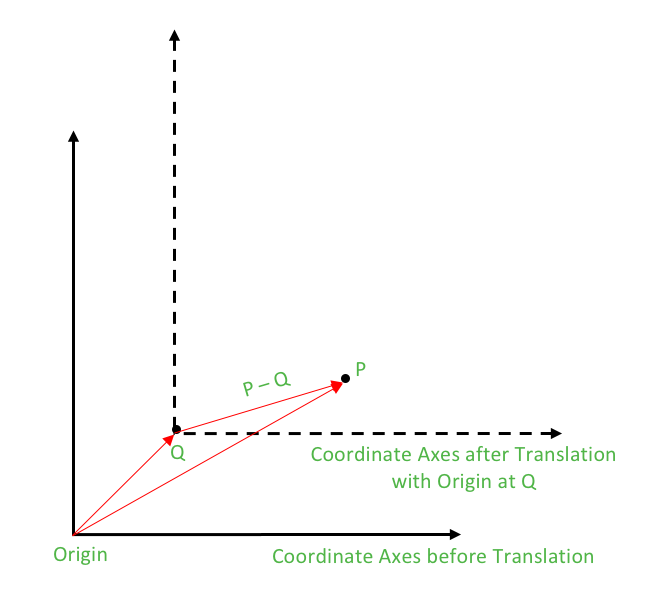# Rotation of a point about another point in C++

• Last Updated : 22 Jun, 2022

We have already discussed the rotation of a point P about the origin in the Set 1 and Set 2. The rotation of point P about origin with an angle θ in the anti-clockwise direction is given as under:

`Rotation of P about origin: P * polar(1.0, θ)`

Rotation of P about point Q

Now, we have to rotate the point P not about origin but about a general point Q. This can be easily understood by the method of translation which is quite a common technique adopted in geometric analysis. What is Translation? In Euclidean geometry, translation is a geometric transformation that moves every point of a figure or a space by the same amount in a given direction. How to Perform Translation? Translation can also be interpreted as the addition of a constant vector to every point, or as shifting the origin of the coordinate system. After the translation, required computations are made and the translation is nullified by subtracting the constant vector to every point or shifting the origin back.So, for rotating P about Q, we shift the origin at Q i.e. we subtract the vector equivalent of Q from every point of the coordinate plane. Now the new point P – Q has to be rotated about the origin and then translation has to be nullified. These steps can be described as under:

1. Translation (Shifting origin at Q): Subtract Q from all points. Thus, P becomes P – Q
2. Rotation of (P – Q) about origin: (P – Q) * polar(1.0, θ)
3. Restoring back the Origin: Add Q to all the points.

Thus,

` Rotation of P about Q : (P – Q) * polar(1.0, θ) + Q`

## CPP

 `// CPP example to illustrate the rotation``// of a point about another point``#include ``#include ` `using` `namespace` `std;` `typedef` `complex<``double``> point;``#define x real()``#define y imag()` `// Constant PI for providing angles in radians``#define PI 3.1415926535897932384626` `// Function used to display X and Y coordinates of a point``void` `displayPoint(point P)``{``    ``cout << ``"("` `<< P.x << ``", "` `<< P.y << ``")"` `<< endl;``}` `//Function for Rotation of P about Q by angle theta``point rotate(point P, point Q, ``double` `theta)``{``    ``return` `(P-Q) * polar(1.0, theta) + Q;``}` `int` `main()``{``    ``// Rotate P about Q``    ``point P(4.0, 3.0);``    ``point Q(2.0, 2.0);` `    ``// Angle of rotation = 90 degrees``    ``double` `theta = PI/2;``    ` `    ``point P_rotated = rotate(P, Q, theta);``    ``cout << ``"The point P on rotating 90 degrees anti-clockwise about Q becomes:"``;``    ``cout << ``"P_rotated"``; displayPoint(P_rotated);` `    ``return` `0;``}`

Output:

`The point P on rotating 90 degrees anti-clockwise about Q becomes: P_rotated(1, 4)`

Time Complexity: O(1)

Auxiliary Space: O(1)

This article is contributed by Aanya Jindal. If you like GeeksforGeeks and would like to contribute, you can also write an article using write.geeksforgeeks.org or mail your article to review-team@geeksforgeeks.org. See your article appearing on the GeeksforGeeks main page and help other Geeks. Please write comments if you find anything incorrect, or you want to share more information about the topic discussed above.

My Personal Notes arrow_drop_up